Function Repository Resource:

# CPDecomposition

Compute the canonical polyadic (CP) decomposition of a tensor

Contributed by: Nikolay Murzin
 ResourceFunction["CPDecomposition"][tensor] returns a list of matrix factors decomposing tensor. ResourceFunction["CPDecomposition"][tensor,rank] provides a positive integer rank for decomposing tensor. ResourceFunction["CPDecomposition"][…,property] returns a property or a list of properties of the decomposition. ResourceFunction["CPDecomposition"][…,All] returns an association with all the properties.

## Details and Options

The CP (canonical polyadic) decomposition is also known as the CANDECOMP/PARAFAC decomposition.
The CP decomposition expresses a tensor as the sum of rank-1 tensors, which can be useful for dimensionality reduction, compression, and revealing latent structure in data.
Given an input tensor and a desired tensor rank, ResourceFunction["CPDecomposition"] returns a list of factor matrices representing the decomposed tensor.
In ResourceFunction["CPDecomposition"][tensor,rank], if rank, the second dimension of each factor, is set to Automatic, the default rank is heuristically determined as Min[16,Total[dims]-Max[dims]], where dims represents the dimensions of the input tensor.
ResourceFunction["CPDecomposition"] uses the alternating least squares (ALS) algorithm to compute the decomposition.
The ALS algorithm is a randomized algorithm. Due to this, ResourceFunction["CPDecomposition"] may return different results on different evaluations. Use SeedRandom or provide "InitialFactors" for reproducibility.
The argument property can be any of the following:
 "Factors" list of matrix factors decomposing an input tensor "ReconstructedTensor" reconstructed tensor "Error" total squared error between input and reconstructed tensors "Iterations" number of iterations performed "Rank" rank of the decompostion
ResourceFunction["CPDecomposition"] takes the following options:
 MaxIterations 100 maximum number of iterations for the ALS algorithm Tolerance 10-6 tolerance threshold for convergence "InitialFactors" Automatic initial list of factors for the ALS algorithm
In the setting of the "InitialFactors" option, the ith factor should have dimensions equal to {di,rank} with di being the tensor’s ith dimension.

## Examples

### Basic Examples (1)

Compute the CP decomposition of a random rank-3 tensor:

 In:=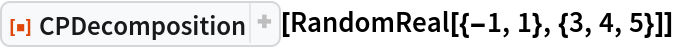Out=### Scope (4)

Return all the properties of the CP decomposition:

 In:=Out=Return a single property:

 In:=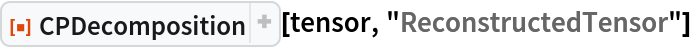Out=Specify a different decomposition rank:

 In:=Out=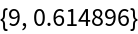Increasing the rank reduces the error:

 In:=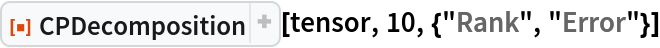Out=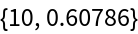Scalars (rank-0 tensors) and vectors (rank-1 tensors) are returned as their own decomposition:

 In:=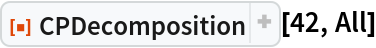Out=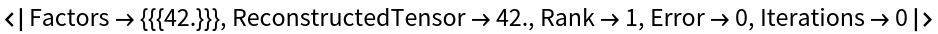In:=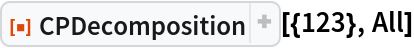Out=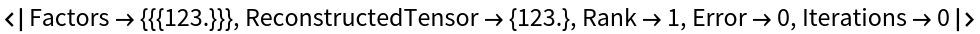CP decomposition of a sparse tensor:

 In:=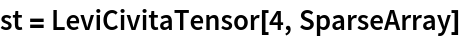Out=In:=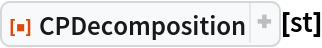Out=### Options (4)

#### InitialFactors (1)

Providing explicit initial factors for the ALS algorithm will make the decomposition deterministic:

 In:=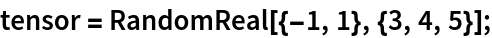In:=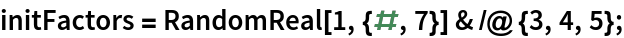In:=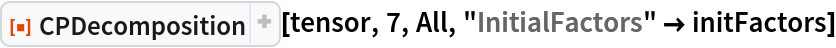Out=#### MaxIterations (1)

Increasing MaxIterations can further reduce the error, at the cost of longer evaluation times:

 In:=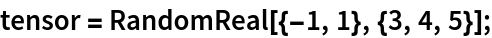In:=Out=In:=Out=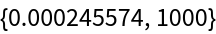#### Tolerance (2)

Increasing the error tolerance will result in faster convergence:

 In:=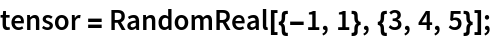In:=Out=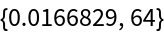Decreasing the error tolerance may reduce error:

 In:=Out=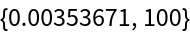### Applications (4)

A 3D test image:

 In:=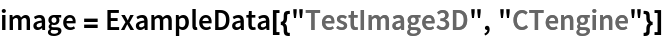Out=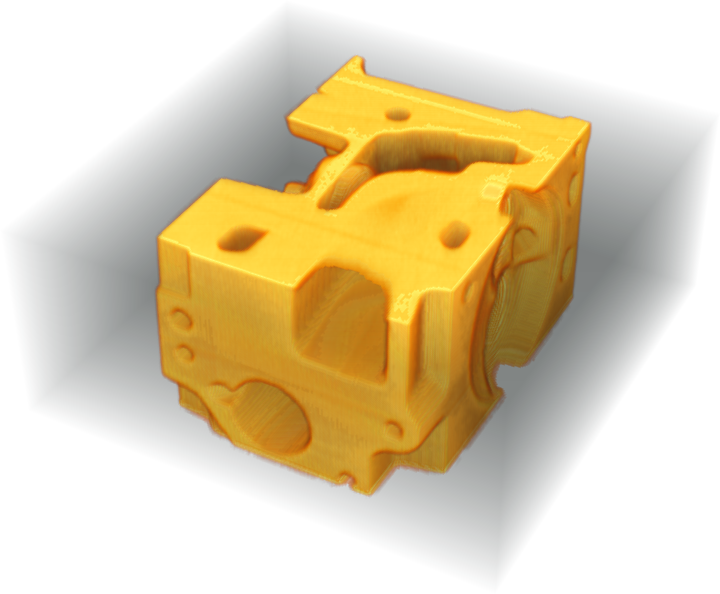Compute the CP decomposition of the image (this might take a long time to evaluate):

 In:=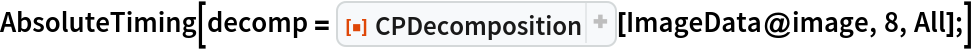Out=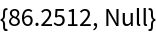The CP decomposition is able to compress the data in the image:

 In:=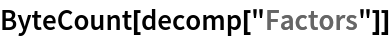Out=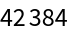In:=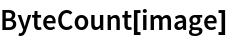Out=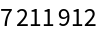Show the reconstructed tensor as an image:

 In:=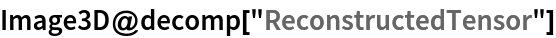Out=### Properties and Relations (2)

Given the CP decomposition factors:

 In:=Out=One can reconstruct a tensor using the ResourceFunction KhatriRaoProduct along with Total and ArrayReshape with initial tensor dimensions:

 In:=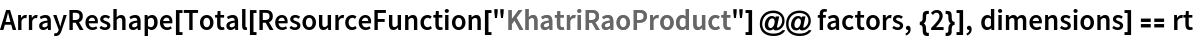Out=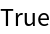The error can be computed using SquaredEuclideanDistance with the flattened original and reconstructed tensors:

 In:=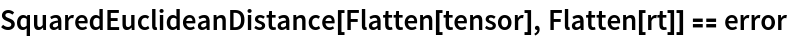Out=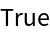For a matrix, CPDecomposition returns two factor matrices a and b, with the original matrix reconstructed as a.Transpose[b]:

 In:=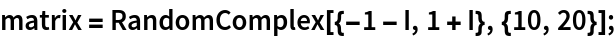In:=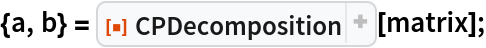In:=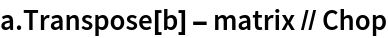Out=## Version History

• 1.0.0 – 05 April 2023